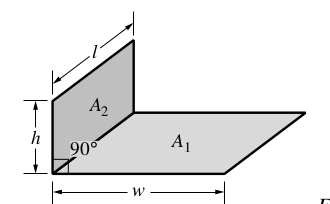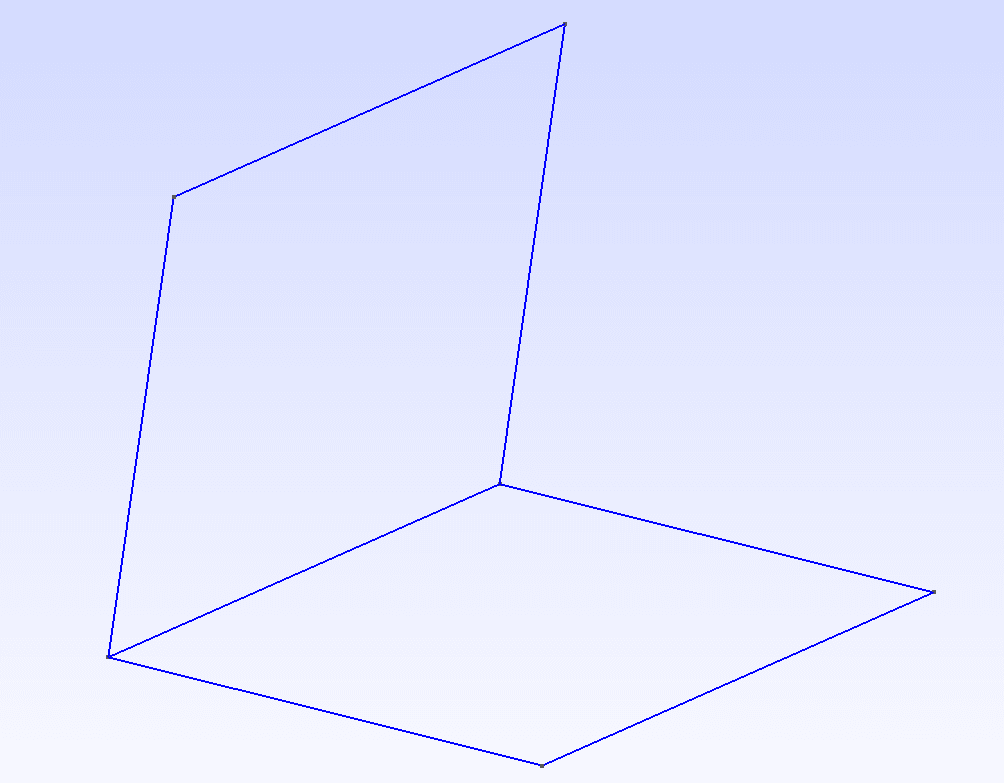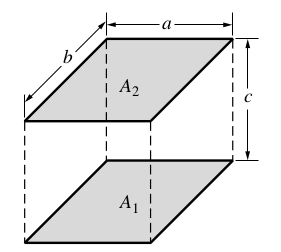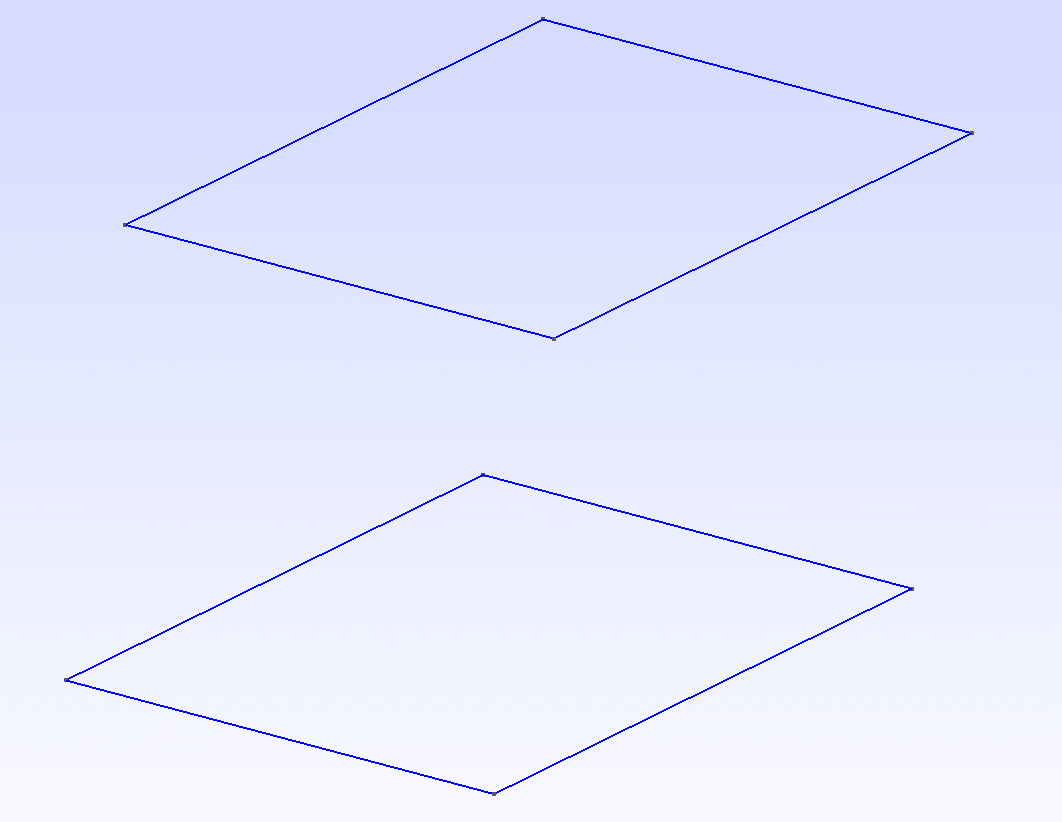# Benchmarks

## 1. Introduction

This section details the benchmarks of calculation of view factor. To see the formula see View Factor

Two examples are use :

• walls which form an right angle

• parallele walls

The during of code is measure and the result is compared with the theorical exact value (see…​).

## 2. Angle of Walls

### 2.1. PresentationFigure 1. Geometrie of angle of wall

The geometry of this benchmarks is two walls wich form an right angle with dimension :

• length w = 1

• height h = 1

• depth l = 1Figure 2. gmsh of geometrie

### 2.2. Execution

To execute angle_walls.cpp :

• Compile with *g* : g angle_walls.cp -o angle_walls

• Execute : ./angle_walls N_s N_r (N_s is the number of source point generated and N_r the number of emissive ray generated by one source)

### 2.3. Results

The results are (with $N_s=1000$, $N_r=1000$) :

• theorical value : $0.222937$

• monte carlo method value : $0.089387$

The during of method is $17356572 ms$

PROBLEM : the result with monte carlo method isn’t the expected result.

## 3. Parallel Walls

### 3.1. PresentationFigure 3. Geometrie of parallel wall

The geometry of this benchmarks is two parallel walls with dimension :

• length a = 1

• width b = 1

• height c = 1Figure 4. Geometrie of parallel wall

### 3.2. Execution

To execute parallel_walls.cpp :

• Compile with *g* : g parallel_walls.cp -o parallel_walls

• Execute : ./parallel_walls N_s N_r (N_s is the number of source point generated and N_r the number of emissive ray generated by one source)

### 3.3. Results

The results are (with $N_s=1000$, $N_r=1000$) :

• theorical value : $0.199825$

• monte carlo method value : $0.131721$

The during of method is $282236 ms$

PROBLEM : the result with monte carlo method isn’t the expected result.